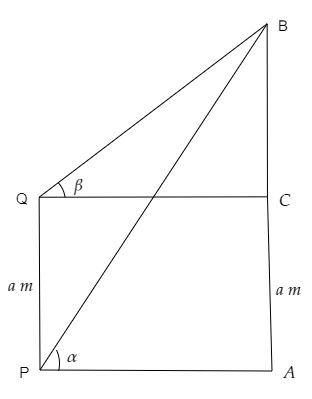# PQ is a post of given height a , and A B is a tower at some distance. If $\alpha$ and $\beta$ are the angles of elevation of B , the top of the tower, at P and Q respectively. Find the height of the tower and its distance from the post.

Given:

PQ  is a post of given height a, and AB  is a tower at some distance. $\alpha$ and $\beta$ are the angles of elevation of  B, the top of the tower, at P and Q respectively.

To do:

We have to find the height of the tower and its distance from the post.

Solution:$PQ$ is the post and $AB$ is the tower.

Angles of elevation of $B$ from $P$ and $Q$ are $\alpha$ and $\beta$ respectively.
$\mathrm{PQ}=a$

Let $\mathrm{AB}=h$ and the distance between the tower and the post be $PA=x\ m$.

$\mathrm{AC}=a$ and $\mathrm{BC}=h-a$

In $\Delta \mathrm{BPA}$,

$\tan \alpha=\frac{\mathrm{BA}}{\mathrm{PA}}$

$=\frac{h}{x}$

$x \tan \alpha=h$.............(i)

Similarly,

In $\Delta \mathrm{BQC}$,

$\tan \beta=\frac{\mathrm{BC}}{\mathrm{QC}}$

$=\frac{h-a}{x}$

$\Rightarrow x \tan \beta=h-a$

$\Rightarrow x \tan \beta=x \tan \alpha-a$                [From (i)]

$\Rightarrow x \tan \alpha-x \tan \beta=a$

$\Rightarrow x(\tan \alpha-\tan \beta)=a$

$\Rightarrow x=\frac{a}{\tan \alpha-\tan \beta}$

$\Rightarrow h=x \tan \alpha=\frac{a}{\tan \alpha-\tan \beta} \times \tan \alpha$

$=\frac{a \tan \alpha}{\tan \alpha-\tan \beta}$

Therefore, the height of tower is $\frac{a \tan \alpha}{\tan \alpha-\tan \beta}$ and the distance between the post and the tower is $\frac{a}{\tan \alpha-\tan \beta}$.

Updated on: 10-Oct-2022

60 Views